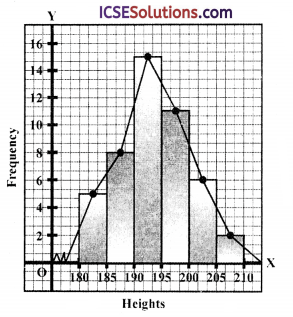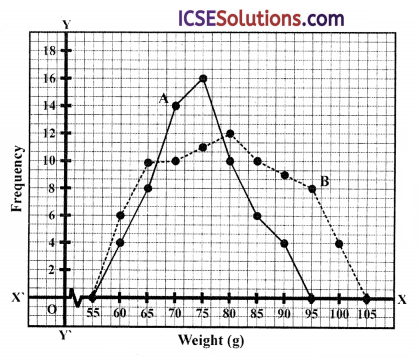The availability of step-by-step S Chand Class 9 Maths Solutions ICSE Chapter 15 Mean, Median and Frequency Polygon Chapter Test can make challenging problems more manageable.

## S Chand Class 9 ICSE Maths Solutions Chapter 15 Mean, Median and Frequency Polygon Chapter Test

Question 1.
The distance (in km) of 40 female engineers from their residence to their place of work were found as follows:

 5 3 10 20 25 11 13 7 12 31 19 10 12 17 18 11 32 17 16 2 7 9 7 8 3 5 12 15 18 3 12 14 2 9 6 15 15 7 6 12

Construct a grouped frequency distribution table with class size 5 for the data given above taking the first interval as 0 – 5 (5 not included). What main features do you observe from this tabular representation?
Solution:Main features :

• The residence of 22 female engineers are with in 5 to 15 i.e., 5 to 10 and 10 to 15 km.
• The residence of 4 female engineers are with in 20 to 35 km (i.e., 20 – 25, 25 – 30, 30 – 35)

Question 2.
The table shows a frequency distribution of the life times of 400 radio tubes tested at a company.
With reference to this table determine the
(i) upper limit of the fifth class.
(ii) lower limit of the eighth class.
(iii) class mark of the seventh class.
(iv) class boundaries of the last class.
(v) class interval size.
(vi) frequency of the fourth class.

 Life time (hours) Number of tubes Life time (hours) Number of tubes 300-399 14 800 – 899 62 400 – 499 46 900 – 999 48 500-599 58 1000 – 1099 22 600 – 699 76 1100- 1199 6 700 – 799 68

Solution:
From the given table,
(i) upper limit of the fifth class is 799
(ii) lower limit of the eighth class is 1000
(iii) class mark of the seventh class = $$\frac{900+999}{2}=\frac{1899}{2}$$ = 949.5
(iv) class boundaries of the last class = 1100 – 1199
(v) class interval size = 100 hours
(vi) frequency of the fourth class = 76Question 3.
What is the median of the values 11, 7, 6, 9, 12, 15, 19
(a) 9
(b) 11
(c) 12
(d) 15
Solution:
Arranging in descending order : 6, 7, 9, 11, 12, 15, 19
Here N = 7
Median = $$\frac { 7+1 }{ 2 }$$ th value = 4th value = 11

Question 4.
The following observations have been arranged in ascending order. If the median of the data is 63, the values of x is
29, 32, 48, 50, x, x + 2, 72, 78, 84, 95
(a) 63
(b) 62.5
(c) 62
(d) 70
Solution:
Median = 63
Observation are in ascending data : 29, 32, 48, 50, x, x + 2, 72, 78, 84, 95
Here N = 10
∴ Median = $$\frac{1}{2}\left[\frac{10}{2} \mathrm{th}+\left(\frac{10}{2}+1\right)\right.$$th observations]
= $$\frac { 1 }{ 2 }$$ [5th + 6th] observation
= $$\frac { 1 }{ 2 }$$ (x + x + 2)
= $$\frac { 2x+2 }{ 2 }$$ = x + 1
∴ x + 1 = 63 ⇒ x = 63 – 1 = 62
Hence, x = 62

Question 5.
(i) The median of the first 10 prime numbers is
(a) 10
(b) 11
(c) 12.5
(d) 12
(ii) The mean of the factors of 36 is
(a) 11
(b) 12$$\frac { 1 }{ 6 }$$
(c) 10$$\frac { 1 }{ 9 }$$
(d) 10$$\frac { 1 }{ 8 }$$
Solution:
(i) First 10 prime numbers in order are : 2, 3, 5, 7, 11, 13, 17, 19, 23, 29
Here N = 10
Median = $$\frac{1}{2}\left[\left(\frac{10}{2} \mathrm{th}\right)+\left(\frac{10}{2}+1 \mathrm{th}\right)\right]$$
= $$\frac { 1 }{ 2 }$$ (5th + 6th) term
= $$\frac { 1 }{ 2 }$$ (11 + 13) = $$\frac { 1 }{ 2 }$$ x 24 = 12

(ii) Factors of 36 are : 1, 2, 3, 4, 6, 9, 12, 18, 36 1 + 2 + 3 + 4 + 6 + 9 + 12 + 18 + 36
∴ Mean = $$\frac{1+2+3+4+6+9+12+18+36}{9}$$ (Here n = 9)Question 6.
A discontinuous distribution of integral data is given below. Draw a frequency polygon.

 Class Interval Frequency 11-20 25 21-30 12 31-40 10 41 -50 15 51-60 8

Solution:

 Class Interval Mid-value Frequency 11-20 15.5 25 21-30 25.5 12 31-40 35.5 10 41 -50 45.5 15 51-60 55.5 8

Taking points (15.5, 25), (25.5, 12), (35.5, 10), (45.5, 15) and (55.5, 8) on the graph and by joining them in order, we get a frequency polygon as given below:Question 7.
The score of a batsman in 25 innings is as under:
48, 25, 110, 65, 12, 10, 40, 45, 90, 14, 24, 48, 55, 5, 1, 17, 80, 20, 18, 30, 59, 115, 9, 49, 17.
(i) Construct a classified frequency distribution table with overlapping class intervals, 0 – 20, 20 – 40, etc.
(ii) Draw a frequency polygon to display the distribution.
Solution:
48, 25, 110, 65, 12, 10, 40, 45, 90, 14, 24, 48, 55, 5, 1, 17, 80, 20, 18, 30, 59, 115, 9, 49, 17

 Class Interval Mid-value Frequency 0-20 10 9 20-40 30 4 40-60 50 7 60-80 70 1 80-100 90 2 100-120 110 2 Total 25

Plotting the points (10, 9), (30, 4), (50, 7), (70, 1), (90, 2) and (110, 2) on the graph.
Here is given its frequency polygon.Question 8.
The members of several professional basketful teams were measured for their heights. The results were:

 Height Frequency 180 ≤ h < 185 5 185 ≤ h < 190 8 190 ≤ h < 195 15 195 ≤ h < 200 11 200 ≤ h <  205 6 205 ≤ h < 210 2

Draw a bar chart and a frequency polygon to illustrate this date.
Solution:

 Height Mid value Frequency 180- 185 182.5 5 185-190 187.5 8 190-195 192.5 15 195-200 197.5 11 200-205 202.5 6 205 -210 207.5 2

(i) Bar chart (Histogram) and frequency polygon is given below:Question 9.
Consider the following data :

 X l 2 3 4 5 y 3 5 9 – 2

The arithmetic mean of the above distribution is 2.96. What is the missing frequency?
(a) 4
(b) 6
(c) 7
(d) 8
Solution:
Mean = 2.96

 X y x × y 1 3 3 2 5 10 3 9 27 4 X 4x 5 2 10 Total 19 + x 50 + 4x

Mean = $$\frac{x y}{f}=\frac{50+4 x}{19+x}$$ = 2.96
50 + 4x = (19 + x) x 2.96
50 + 4x = 56.24 + 2.96x
⇒ 4x – 2.96x = 56.24 – 50 = 6.24
⇒ 1.04x = 6.24
⇒ x = $$\frac { 6.24 }{ 1.04 }$$
∴ x = 6Question 10.
Two frequency polygons are shown giving the distribution of the weights of players in two different sports A and B.(i) How many people played sport A?
(ii) Comment on two differences between the two frequency polygons.
(ii) Either for A or for B suggest a sport where you would expect the frequency polygon of weights to have this shape. Explain in one sentence why you have chosen that sport.
Solution:
(i) Number of people who play sport A = 4 + 8 + 14 + 16 + 10 + 6 + 4 = 62

(ii) Sport B has more heavy people. Sport A has much smaller range of weights compared to sport B which has at the most weight 105 kg and A has 95 kg.

(iii) As per frequency polygons sport A has smaller range of weights, so it must be a game that does not require heavy weight of the player. Sport A can be a cricket or badminton. Since sport B has heavy people, so Kabbadi, wrestling or weight lifting like games can be suggested for sport B.